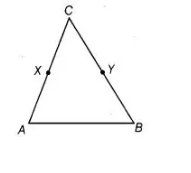`
Question:

In the adjoining figure, we have X and Y are the mid-points of AC and BC and AX = CY. Show that AC = BC.Solution:

Given, X is the mid-point of AC

AX = CX = ½ AC

=> 2AX =2CX = AC ……(i)

and Y is the mid-point of BC.

BY =CY = ½ BC

=> 2BY=2CY = BC …(ii)

Also, given AX=CY …(iii)

According to Euclid’s axiom, things which are double of the same things are equal to one another.

From Eq. (iii), 2AX = 2CY

=> AC = BC [from Eqs. (i) and (ii)]